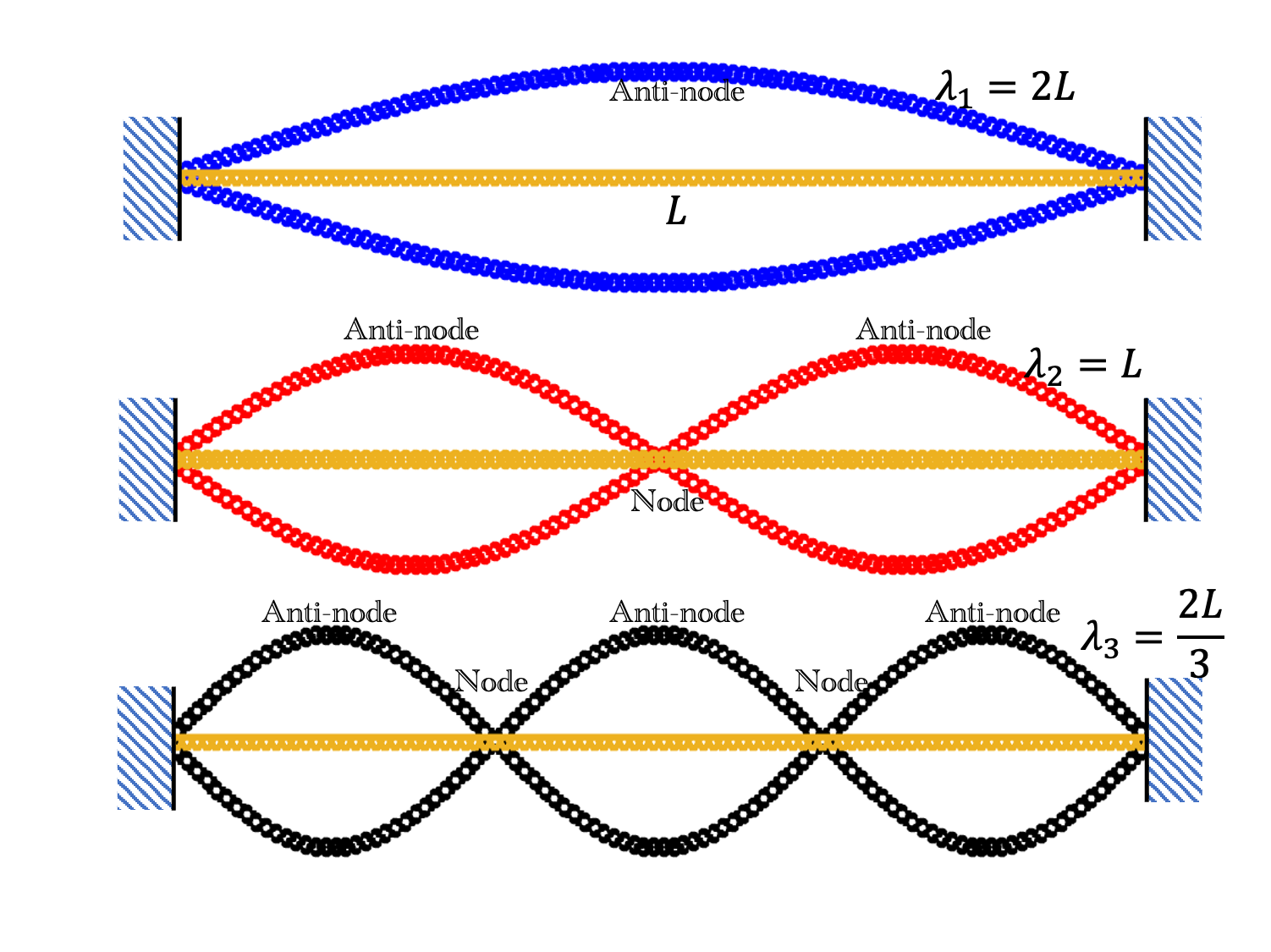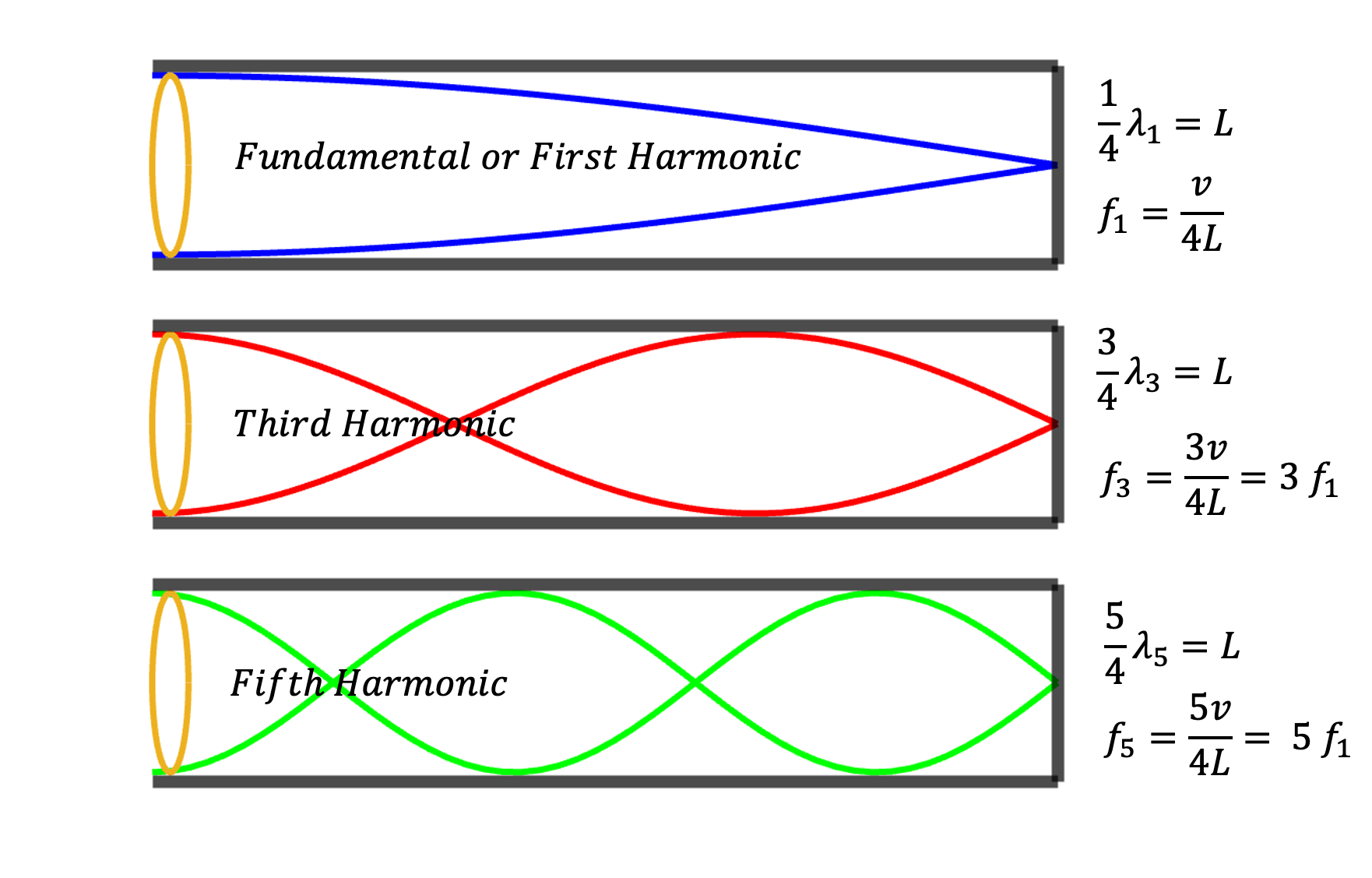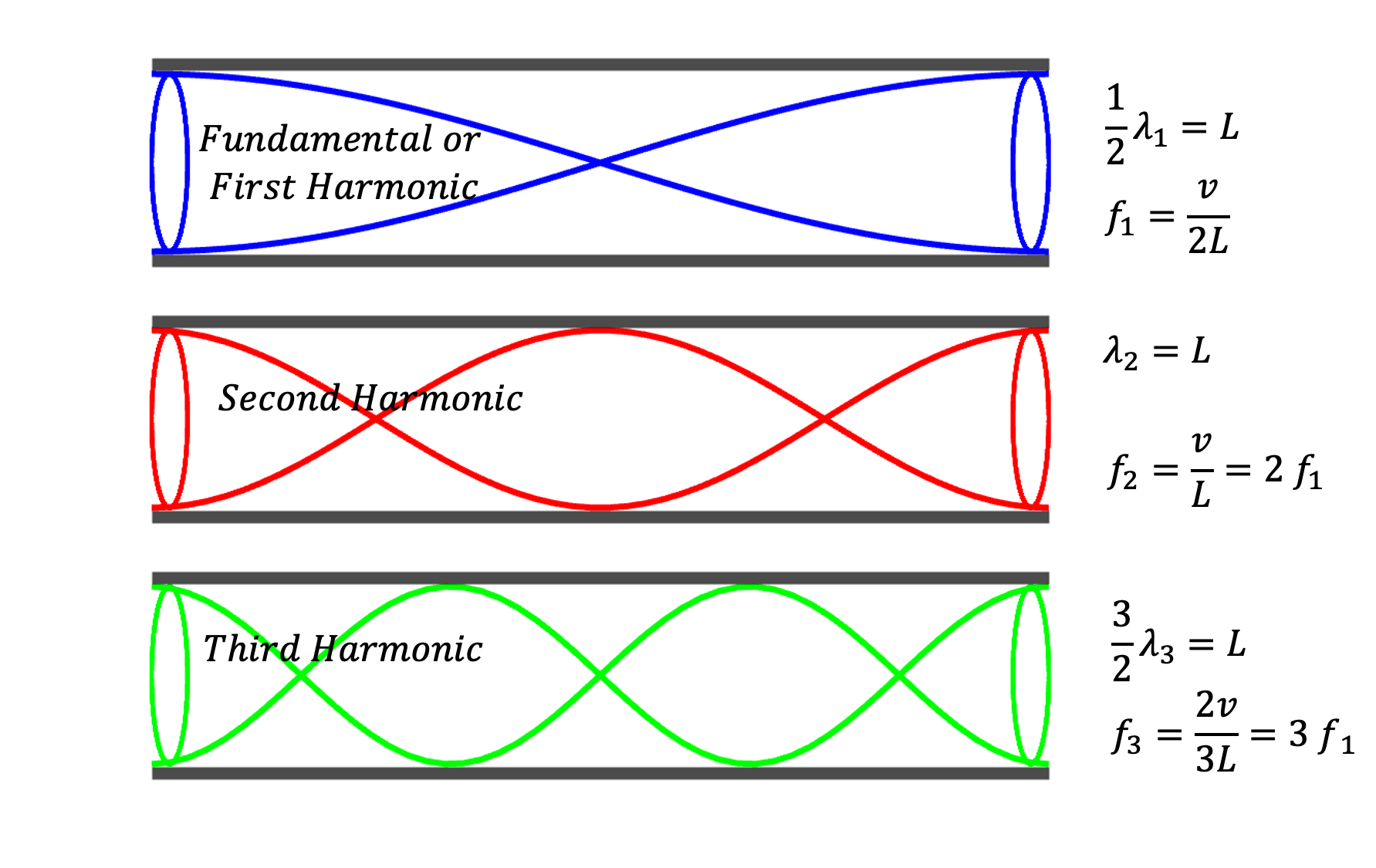## Section15.5Musical Instruments

All sounds have their source in vibration. When you pluck a guitar string, you make it vibrate which, in turn, vibrates air particles causing sound wave to travel through air to our ears. Vibrations on strings of a violin are excited by rubbing on the string by a bow. Vibrations on a drum are excited by hitting the taut membrane. In wind instruments usuallt a column of air is set to vibrate by a vibrating reed or vibrating lip of a player or just blowing over an opening. In all cases, vibrations set up standing waves. We will examine them below.

### Subsection15.5.1Stringed Instruments

We can understand sound produced by stringed instruments such as guitar, piano, violin, etc, in terms of normal modes of each string as discussed in Subsection 14.3.2. First three harmonics, i.e., the fundamental, first overtone, and second overtone are shown in Figure 15.5.1.Figure 15.5.1. Normal modes of a string tied at both ends. The figure shows modes of lowest three frequencies. These modes are teh first three harmonics or the fundamental, the first overtone, and the second overtones. The wavelengths are $\lambda_1 = 2 L\text{,}$ $\lambda_2 = L\text{,}$ and $\lambda_3 = \frac{2L}{3}\text{.}$ The locations of maximum displacment are called anti-nodes and the locations of zero displacement are called nodes.

You can tell that the wavelengths of normal modes of a string of length $L$ tied at both ends are given by

\begin{equation*} \lambda_1 = 2 L,\ \lambda_2 = L,\ \ \lambda_3 = \frac{2L}{3},\ \cdots. \end{equation*}

With $v$ denoting speed of waves on a string, the corresponding frequencies are

\begin{equation*} f_1 = \frac{v}{\lambda_1}, \ f_2 = \frac{v}{\lambda_2} = L,\ \ f_3 = \frac{v}{\lambda_3},\ \cdots. \end{equation*}

Wave speed depend on the mass per unit length $\mu$ and tension $T$ of the string.

\begin{equation*} v = \sqrt{\frac{T}{\mu}}. \end{equation*}

You can see that you need to change $L$ to change wavelength, but, you can change frequency by changing speed $v\text{,}$ which can be increased by increasing tension or using a string with less mass per unit length. If $v$ goes up, the frequency will go up.

### Subsection15.5.2Wind Instruments

Wind instruments such as flute, clarinet, trumpet, etc, rely on setting up standing sound waves in an air column by blowing over an end, vibrating a membrane, vibrating lips, vibrating a reed, etc.

A simple way to study these instruments is to understand modes of air vibrations in a simple tube, which can be three types - (i) open at one end and closed at the other end, (ii) open at both ends, and (iii) closed at both ends. Displacement of air particles will be zero at the close ends and will be maximum at the open ends.

Using the rule about displacments at open ends and at close ends we can actually draw pictures of pieces of sine function that would fit in the tube we can guess wavelengths of the modes as illustrated in Figure 15.5.2.Figure 15.5.2. Lowest frequency displacment modes of resonance in an air column that is open at one end and closed at the other. In terms of the length $L$ of the tube, we see that only a quarter of the wave fits in the tube with largeest displacment (antinode) at the opening and zero displacment (node) at the close end. Therefore, wavelength corresponding to this mode will be $\lambda = 4 L\text{.}$ With $v$ the wave speed, the corresponding frequency will be $f_1 = v/\lambda_1 = b/4L\text{.}$ We work out wavelength and frequency of other modes similarly. Try to guess the fourth mode that will be excited in this tube.

If you were to look at pressure wave in the tube in Figure 15.5.2, you will find that the roles of nodes and antinodes switch - the place where there is a node in displacement wave, you will find antonode, i.e., highest pressure difference at that point and at the points of antinode in the displacement wave, you will have nodes in the pressure wave. It makes sense that at the opening of a tube, you should have a node in the pressure wave since you shoulf find pressure to be same as the ambient pressure, and zero pressure difference from the ambient.

If both ends of a tube are open, the displacement at the two ends will take largest amplitude there. As shown in Figure 15.5.3 Fitting pieces of sine or cosine functions, we find that the lowest mode, i.e., the fundamental mode, will have half a wave length of a sine wave. Therefore, the fundamental has wavelength, $\lambda_1 = 2L$ and the fundamental frequency $f_1=v/\lambda_1 = v/2L\text{.}$ Similarly, we fit the overtones. Here, we find that, unlike one end-open-other-end-closed system, here we have all integral multiples of the fundamentl frequencies. While in Figure 15.5.2 we had only third, fifth, etc., here we have second, third, fourth, etc., all of them.Figure 15.5.3. Lowest frequency displacment modes of resonance in an air column that is open at both ends. In terms of the length $L$ of the tube, we see that only a half of the wave fits in the tube with largest displacment (antinode) at the ends - we necessarily must have one node in the middle of the tube. Therefore, wavelength corresponding to this mode will be $\lambda = 2 L\text{.}$ With $v$ the wave speed, the corresponding frequency will be $f_1 = v/\lambda_1 = b/2L\text{.}$ We work out wavelength and frequency of other modes similarly. Try to guess the fourth mode that will be excited in this tube.